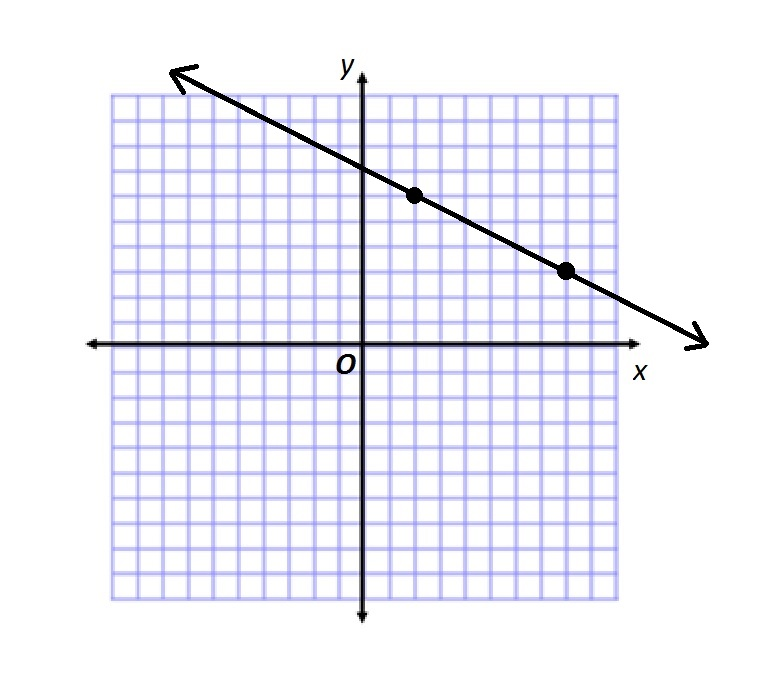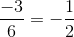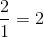# PSAT Math : How to find the slope of perpendicular lines

## Example Questions

2 Next →

### Example Question #61 : GeometryGive the slope of a line perpendicular to the line in the above figure.None of the other responses is correct.Explanation:

In order to move from the upper left point to the lower right point, it is necessary to move down 3 units and right six units. This is a rise ofand a run of 6. The slope of a line is the ratio of rise to run, so slope of the line shown is.

A line perpendicular to this will have a slope equal to the opposite of the reciprocal of. This is.

2 Next →

### All PSAT Math Resources